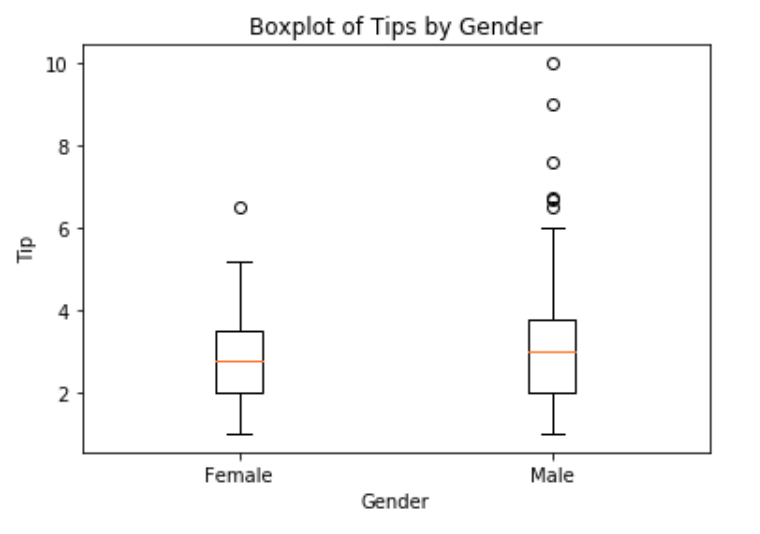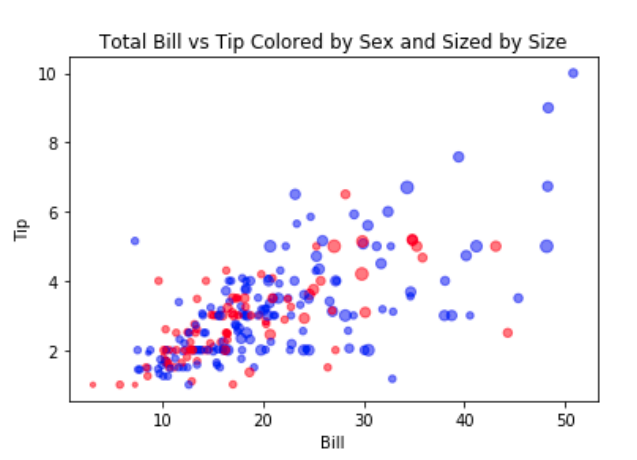top of page
Search

# Statistical Data Visualization Assignment Help | Types of Data Visualization | Realcode4you

In a visualisation process, we use different types of graphs to present information for different types of data.

Data could be differentiated by the number of variables in the dataset.

When there is only one variable in the dataset or function, it is known as univariate.

A dataset, expression, function or statistical models with two variables is called bivariate.

A multivariate dataset contains many variables.

Univariate

Histograms are the most common means of looking at a single variable. The diagram consists of rectangles whose area is proportional to the frequency of the variable and whose width is equal to the class interval. Also, in a histogram, we group the values together (i.e. they are “binned”) which are then plotted to show the distribution of the variables.

We will use the tip data from the seaborn library to plot our histogram. The tip data contains the amount of the tips that people leave, with various variables such as total cost of bill, size (number of diners), day of the week, etc.

```import seaborn as sns

output:We can use the matplotlib.pyplot library to construct the histogram as shown below. In this example, we divide the frequency of the data set into 10 interval (bins):

```# this line required for matplot to work in
# jupyter notebook
%matplotlib inline
import matplotlib.pyplot as plt
fig = plt.figure()
axes1.hist(tips['total_bill'],bins=10)
axes1.set_xlabel('Total Bill')
axes1.set_ylabel('Frequency')```

output:We can see from the graph that the most common tip given by customers is around \$15- \$20 - as there are more than 65 such tips given.

If we change the bin size to say 100, then we can see even more granular details of the number of tips given at different tip amount.Bivariate

We demonstrate here two types of plots to present two variables.

Scatterplot

We use a scatterplot to plot one continuous variable against another continuous variable.

```%matplotlib inline
import matplotlib.pyplot as plt
fig = plt.figure()
axes1.scatter(tips['total_bill'],tips['tip'])
axes1.set_title('Scatterplot of Total Bill vs Tip')
axes1.set_xlabel('Total Bill')
axes1.set_ylabel('Tip')```

out:Boxplot

We use Boxplot to plot a discrete variable against a continuous variable. In our example, sex is a kind of discrete variable, as it is either Male or Female.

```%matplotlib inline
import matplotlib.pyplot as plt
fig = plt.figure()
axes1.boxplot(
# first argument of boxplot is the data
# we put each piece of data into a list here
[tips[tips['sex'] == 'Female']['tip'],
tips[tips['sex'] == 'Male']['tip']],
# we pass an optional labels parameter here
labels=['Female', 'Male']
)
axes1.set_title('Boxplot of Tips by Gender')
axes1.set_xlabel('Gender')
axes1.set_ylabel('Tip')
fig.show()

```

output:The boxplot shows that the median (orange horizontal line) tip for Male is slightly higher than for Female. The box represents the Interquartile Range (IQR), which is the 25 percentile to 75 percentile of the data. The circles represent data points that are outside the wide margins of the boxplots. They are also known as outliers. You can check out this link here for some excellent comments on how to interpret the boxplot:

https://stackoverflow.com/questions/17725927/boxplots-in-matplotlib-markers-and-outliers

Multivariate

Data There is no standard method to present multivariate data, since each case could be unique.

To illustrate the process of plotting multivariate data, let’s build on the scatterplot earlier

We have two variables: tip and total bill.

To add another variable, say gender, one option is to colour the data points based on the value of the third variable.

If we want to add a fourth variable, say size, we could show this by adjusting the size of the dot representing each data point.

There are some practical points that we need to consider when using colours and size. Human eyes cannot really perceive small differences in size, and many dots add clutter to the visualisation. One technique to reduce clutter is to add some value of transparency to the individual points, such that many overlapping points will show a darker region of plot than less crowded areas.

While colours are much easier to distinguish than changes in size, the choice of colour palettes might not be easy as we cannot perceive hues on a linear scale.

Luckily matplotlib and seaborn come with their own set of colour palettes, and tools like colorbrewer can help with the colour picking process.

The following codes use colour to add a third variable, sex, and set the size of the dots to represent size (number of diners) to our earlier scatter plot.

```# define a method to set color variable based on sex
def recode_sex(sex):
if sex == 'Female':
# red for female
return 'r'
else:
# blue for male
return 'b'
# create a new column, sex_color, by applying the method
# on the existing column, sex
tips['sex_color'] = tips['sex'].apply(recode_sex)
scatter_plot = plt.figure()
axes2.scatter(
x = tips['total_bill'],
y = tips['tip'],

# set the size of the bots based on party size
# we multiply the values by 10 to make the points bigger
# and to emphasize the difference
s = tips['size']*10,

# set the color for the sex
c = tips['sex_color'],
# set the alpha value so that the points will be
transparent
# this helps with overlapping points
alpha=0.5)
axes2.set_title('Total Bill vs Tip Colored by Sex and Sized by
Size')
axes2.set_ylabel('Tip')
axes2.set_xlabel('Bill')
scatter_plot.show()

```

output:Seaborn Plotting Library

The matplotlib library is the foundational plotting tool in Python. The seaborn library builds on matplotlib to provide higher-level interface for statistical graphics. It also provides an interface to produce prettier and more complex visualisations with fewer lines of code.

Let’s go through the different types of plots that could be used to present different types of data types.

Univariate

Data Histograms

We create histogram using sns.distplot

```%matplotlib inline
import matplotlib.pyplot as plt
import seaborn as sns
# The subplots function is a shortcut for
# creating separate figure objects and
# adding individual subplots (axes) to the figure
figure, ax = plt.subplots()
# to display histogram only, set kde=False
ax = sns.distplot(tips['total_bill'], kde=True)
ax.set_ylabel('Frequency')
ax.set_xlabel('Total Bill')
ax.set_title("Total Bill Histogram with Density Plot")```

The default distplot plots both a histogram and a density plot (using a kernel density estimation).Density

Plot Density plot is another way to visualise a univariate distribution. It is created by drawing a normal distribution centred at each data point, and then smoothing out the overlapping plots so that the area under the curve is 1.

Bar Plot

Bar plots are similar to histograms. They are used to count discrete variable. The following codes produce a count plot to see the count of tips given, grouped by sex:

```figure, ax = plt.subplots()
ax = sns.countplot(tips['sex'])```

output:Bivariate Data

Scatterplot

The seaborn library uses regplot to plot a scatterplot, and at the same time fit in a regression line.

```figure, ax = plt.subplots()
ax = sns.regplot(x = 'total_bill', \
y='tip', data=tips , fit_reg=True)
ax.set_title('Scatterplot of Total Bill and Tip')
ax.set_xlabel('Total Bill')
ax.set_ylabel('Tip')```

output:If we set the parameter fit_reg=False,then the regression line will be removed.

Jointplot

We can make a scatterplot to include a univariate plot on each axis, using jointplot:

```joint = sns.jointplot(x = 'total_bill', y='tip', data=tips)
joint.fig.suptitle('Joint Plot of Total Bill and Tip')
joint.set_axis_labels('Total Bill','Tip')```

output:Hexbin Plot

Sometimes, there are too many data points for a scatterplot to be meaningful. One way to get around this issue is to bin points on the plot together. Just as histograms can bin a variable to create a bar, hexbin can bin two variables. A hexagon is used for this purpose because it is the most efficient shape to cover an arbitrary 2D surface:

```joint = sns.jointplot(x = 'total_bill', \
y='tip', data=tips, kind='hex')
joint.fig.suptitle('Hexbin Joint Plot of Total Bill and Tip')
joint.set_axis_labels('Total Bill','Tip')```

output:Boxplot

Boxplots are created using the boxplot function:

```fig, ax = plt.subplots()
ax = sns.boxplot(x='time', y = 'total_bill', data=tips) ```

output:Pairplot

When we have a number of numeric data, we can also quickly visualise all the pairwise relationships using the pairplot function. This function plots a scatterplot between each pair of variables, and a histogram for the univariate data

`fig = sns.pairplot(tips)`

output:Conclusion

Data visualisation is an integral part of data exploration, analysis and presentation. We have provided here an introduction to the various ways to explore and present your data. There are many plotting and visualisation resources available on the internet - these include the seaborn documentation, Pandas visualisation documentation and matplotlib documentation. Other resources include colorbrewer to help pick good colour schemes. The plotting libraries we used in this chapter also have various colour schemes that could be used to adjust your visualisation output. It is important that you know how to reach out to the right resources to get the solutions required for your project.

Realcode4you.com is the right resources where you get all data visualization related help in Python, R studio, Tableau, Power BI, etc.

For more details you can send your request at:

realcode4you@gmail.com